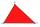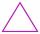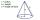QuizQ

An isosceles triangle has two sides of length 7 km and 39 km. How long is a third side?

Result

x =  39 km

Solution:Leave us a comment of example and its solution (i.e. if it is still somewhat unclear...):Be the first to comment!Next similar examples:

1. TriangleFor how many integer values of x can 16, 15 and x be the lengths of the sides of triangle?
2. MidpointsTriangle whose sides are midpoints of sides of triangle ABC has a perimeter 45. How long is perimeter of triangle ABC?
3. 3-bracket 3Two angles in a triangle are 90° and 60°. Has triangle at least two equal sides?
4. 3-bracket 2May be the smallest angle in the triangle greater than 40°?
5. 3-bracketMay be the largest angle in the triangle less than 20°?
6. Triangle P2Can triangle have two right angles?
7. Pizza 4Marcus ate half pizza on monday night. He than ate one third of the remaining pizza on Tuesday. Which of the following expressions show how much pizza marcus ate in total?
8. HotelThe hotel has a p floors each floor has i rooms from which the third are single and the others are double. Represents the number of beds in hotel.
9. NormThree workers planted 3555 seedlings of tomatoes in one dey. First worked at the standard norm, the second planted 120 seedlings more and the third 135 seedlings more than the first worker. How many seedlings were standard norm?
10. Write 2Write 791 thousandths as fraction in expanded form.
11. Equation 29Solve next equation: 2 ( 2x + 3 ) = 8 ( 1 - x) -5 ( x -2 )
12. Dropped sheetsThree consecutive sheets dropped from the book. The sum of the numbers on the pages of the dropped sheets is 273. What number has the last page of the dropped sheets?
13. Unknown number 11That number increased by three equals three times itself?
14. AlleyAlley measured a meters. At the beginning and end are planted poplar. How many we must plant poplars to get the distance between the poplars 15 meters?
15. The diagramThe diagram is a cone of radius 8cm and height 10cm. The diameter of the base is. ..
16. DecideThe rectangle is divided into seven fields. On each box is to write just one of the numbers 1, 2 and 3. Mirek argue that it can be done so that the sum of the two numbers written next to each other was always different. Zuzana (Susan) instead argue that.
17. Find xSolve: if 2(x-1)=14, then x= (solve an equation with one unknown)